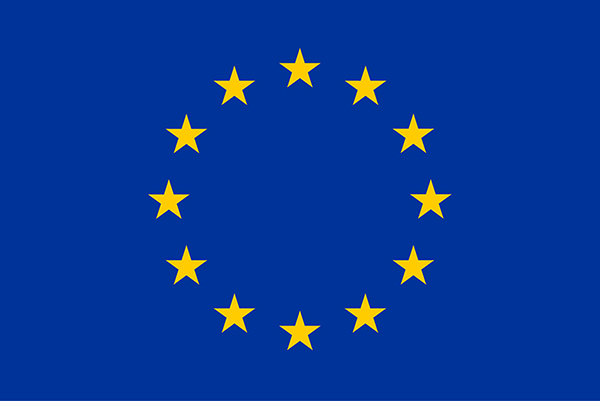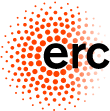# IWOTA 2014

## special session on free probability and operator theory (FPOT)

(Amsterdam, July 14 to July 18, 2014)Funded by the ERC Advanced Grant Non-Commutative Distributions in Free Probability

Organized by Serban Belinschi (Queens), Roland Speicher (Saarbrücken) and Moritz Weber (Saarbrücken).

This homepage provides information about the special session on free probability and operator theory (FPOT),
which is part of the International Workshop on Operator Theory and its Applications (IWOTA).

Our session (all eight talks) is scheduled for Monday afternoon. Note that this is a preliminary schedule.

### Day 1 - Aspects of free analysis related to free probability and operator theory

(Monday, 13.30 - 15.15, room M639)
• Daniel Alpay (Beer-Sheva, Israel)
On free stochastic processes and their derivatives

• Tobias Mai (Saarbrücken, Germany)
On the calculation of distributions and Brown measures

• Victor Vinnikov (Beer-Sheva, Israel)
Noncommutative Hardy classes

• John Williams (Texas A&M, USA)
Computational results in operator-valued free probability

### Day 2 - Aspects of quantum groups and groups related to free probability and operator theory

(Monday, 15.45 - 17.30, room M639)
• Uwe Franz (Besançon, France)
Lévy processes on free compact quantum groups

• François Lemeux (Besançon, France)
Combinatorial and operator algebraic properties for compact quantum groups

• Sven Raum (Lyon, France)
The classification of easy quantum groups

• Andreas Thom (Leipzig, Germany)
Random walks and invariant random forrests

### Abstracts

• Daniel Alpay (Beer-Sheva, Israel)
On free stochastic processes and their derivatives  In the commutative setting, Hida's white noise space gives a framework to construct stochastic processes with stationary increments, and their derivatives exist in the Kondratiev's space of stochastic distributions (see ). This space is in fact a topological algebra, and has a special structure, in terms of the Vage inequalities, which relate the product with the underlying norms. In the talk we discuss the non-commutative version of the above analysis. We first discuss non-commutative spaces of stochastic test functions and distributions. The latter space contains the non-commutative white noise space, and is a topological algebra. See . Then we present a family of free stochastic processes with stationary increments. The values of the derivatives are now continuous operators from the space of stochastic test functions into the space of stochastic distributions. Finally we define a stochastic integral for the given family of free processes. See . The talk is based on joint works with Palle Jorgensen (University of Iowa) and Guy Salomon (Ben-Gurion University).  D. Alpay, H. Attia and D. Levanony. On the characteristics of a class of Gaussian processes within the white noise space setting. Stochastic Processes and their Applications, vol. 120 (issue 7), pp. 1074-1104 (2010).  D. Alpay, P. Jorgensen and G. Salomon, On free stochastic processes and their derivatives. ArXiv e-prints 1311.3239 (2013).  D. Alpay and G. Salomon. Non-commutative stochastic distributions and applications to linear systems theory. Stochastic Processes and Applications, vol. 123 (2013), pp. 2303-2322.

• Uwe Franz (Besançon, France)
Lévy processes on free compact quantum groups  We recall the basic theory of Lévy processes on involutive bialgebras and CQG algebras and discuss recent classification results for Lévy processes on the free orthogonal quantum groups ON+ and the free permutation quantum groups SN+. We also study in more detail some examples of Lévy processes on these quantum groups and on the free unitary quantum group UN+. Based on joint work with Fabio Cipriani, Anna Kula, and Adam Skalski.  F. Cipriani, U. Franz, A. Kula: Symmetries of Lévy processes on compact quantum groups, their Markov semigroups and potential theory. J. Funct. Anal. 266 (2014), no. 5, 2789-2844.  U. Franz, A. Kula, A. Skalski: Lévy processes on quantum symmetry groups, in preparation.

• François Lemeux (Besançon, France)
Combinatorial and operator algebraic properties for compact quantum groups  After recalling a few definitions about Wang's free quantum groups such as free orthogonal and unitary quantum groups and quantum permutation groups, I will give the definition of Bichon for free wreath product quantum groups. I will explain how to compute the fusion rules of certain free wreath products by the quantum permutation groups. To do this I will focus on giving a combinatorial description of the intertwiner spaces in such free wreath products. I will give some applications of the knowledge of such fusion rules such as approximation properties and factoriality.

• Tobias Mai (Saarbrücken, Germany)
On the calculation of distributions and Brown measures  The linearization trick in its self-adjoint version due to G. Anderson allows, in combination with results about the operator-valued free additive convolution, an algorithmic solution to quite general questions in free probability theory, which also have applications in random matrix theory. For instance: (1) How can we compute the distribution of self-adjoint non-commutative polynomials in free random variables? (answered in joint work with S. Belinschi and R. Speicher) (2) How can we compute the Brown measure of non-commutative polynomials in free random variables? (answered by S. Belinschi, P. Sniady, and R. Speicher) In my talk, I will present these solutions and I will discuss, how they can be extended from non-commutative polynomials to the case of non-commutative rational functions.

• Sven Raum (Lyon, France)
The classification of easy quantum groups  Motivated by results in free probability theory and combinatorics, Banica and Speicher introduced in 2009 easy quantum groups. In free probability theory they are used to describe non-classical symmetries of specific distributions. The class of easy quantum groups contains the important free orthogonal quantum group and the quantum permutation group. They were introduced by Wang and constitute original examples of quantum groups, which cannot be obtained by deforming classical groups. However, there are many more easy quantum groups. Contributions to their classification were made by Banica-Speicher, Banica-Curran-Speicher and Weber. Recently it was completed by Raum-Weber. In this talk we introduce the concept of easy quantum groups, demonstrate their connection with free probability and describe their classification. Based on this classification, we point out some further directions of research in this field. The talk is based on joint work with Moritz Weber.

• Andreas Thom (Leipzig, Germany)
Random walks and invariant random forrests  We show that every finitely generated non-amenable group has finite generating sets with arbitrarily small spectral radius. Together with a new estimate for the expected degree for the free minimal spanning forest, this gives some new insight on percolation of non-amenable Cayley graphs - and confirms a weak form of a conjecture due to Itai Benjamini and Oded Schramm. We also give some applications to a characterization of amenability of group von Neumann algebras in terms of operator spaces and apply the methods to particular cases of Dixmiers problem about amenability of unitarisable groups.  A. Thom: The expected degree of minimal spanning forests, to appear in IMRN, arXiv:1306.0303.  A. Thom: A remark about the spectral radius, submitted, arXiv:1306.1767.

• Victor Vinnikov (Beer-Sheva, Israel)
Noncommutative Hardy classes  I will discuss the emerging theory of Hardy classes on the noncommutative unit ball over a finite dimensional operator space with a variety of operator space norms. The methods combine the general theory of nc functions with asymptotic freeness results of D.-V. Voiculescu and formulae for integration over unitary groups of B. Collins and P. Sniady. It is the first step in developing a general theory of nc bounded symmetric domains. This is a joint work with Mihai Popa.

• John Williams (Texas A&M, USA)
Computational results in operator-valued free probability  In the 90's and 2000's, the theory of free probability was extended to operator-valued distributions in order to study amalgamated free product phenomena in operator theory. While much theoretical work has been done in studying this field there are few concrete calculations of the convolution operations and transforms. In this talk, we present several new techniques in calculating the convolution operations (arising from both analytic and combinatorial methods) and several concrete examples of the convolution operation with explicit identification of the moments of the convolved distributions.

Link to the IWOTA 2014 webpage

 Updated: 26 June 2014   Moritz Weber Impressum